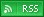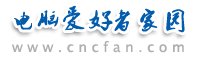电脑爱好者，提供IT资讯信息及各类编程知识文章介绍，欢迎大家来本站学习电脑知识。 最近更新 | 联系我们站内搜索：

# java学习总结-Object 类

JAVA中有一个特殊的类： Object。它是JAVA体系中所有类的父类（直接父类或者间接父类）。

(1)finalize方法：当一个对象被垃圾回收的时候调用的方法。
(2)toString():是利用字符串来表示对象。

(3)equal

String A=new String(“hello”);
String B=new String(“hello”);
A==B(此时程序返回为FALSE)

String a=”hello”;
System.out.println(a==”hello”);

System.out.println(a.equal(b));
equal用来比较两个对象中字符串的顺序。
a.equal(b)是a与b的值的比较。

student a=new student(“LUCY”,20);
student b=new student(“LUCY”,20);
System.out.println(a==b);
System.out.println(a.equal(b));

public boolean equals(Object o){
if (this==o) return trun; //此时两者相同
if (o==null) return false;
if (! o instanceof strudent) return false; //不同类
studeng s=(student)o; //强制转换
if (s.name.equals(this.name)&&s.age==this.age) return true;
else return false;
}
 java热门文章排行 网站赞助商